# griddata

Interpolate 2-D or 3-D scattered data

## Syntax

``vq = griddata(x,y,v,xq,yq)``
``vq = griddata(x,y,z,v,xq,yq,zq)``
``vq = griddata(___,method)``
``[Xq,Yq,vq] = griddata(x,y,v,xq,yq)``
``[Xq,Yq,vq] = griddata(x,y,v,xq,yq,method)``

## Description

example

````vq = griddata(x,y,v,xq,yq)` fits a surface of the form v = f(x,y) to the scattered data in the vectors `(x,y,v)`. The `griddata` function interpolates the surface at the query points specified by `(xq,yq)` and returns the interpolated values, `vq`. The surface always passes through the data points defined by `x` and `y`.```

example

````vq = griddata(x,y,z,v,xq,yq,zq)` fits a hypersurface of the form v = f(x,y,z).```
````vq = griddata(___,method)` specifies the interpolation method used to compute `vq` using any of the input arguments in the previous syntaxes. `method` can be `"linear"`, `"nearest"`, `"natural"`, `"cubic"`, or `"v4"`. The default method is `"linear"`.```
````[Xq,Yq,vq] = griddata(x,y,v,xq,yq)` and `[Xq,Yq,vq] = griddata(x,y,v,xq,yq,method)` additionally return `Xq` and `Yq`, which contain the grid coordinates for the query points.```

## Examples

collapse all

Interpolate random scattered data on a uniform grid of query points.

Sample a function at 200 random points between `-2.5` and `2.5`. The resulting vectors `x`, `y`, and `v` contain scattered sample points and data values at those points.

```rng default xy = -2.5 + 5*rand([200 2]); x = xy(:,1); y = xy(:,2); v = x.*exp(-x.^2-y.^2);```

Define a grid of query points and interpolate the scattered data over the grid.

```[xq,yq] = meshgrid(-2:.2:2, -2:.2:2); vq = griddata(x,y,v,xq,yq);```

Plot the gridded data as a mesh and the scattered data as dots.

```mesh(xq,yq,vq) hold on plot3(x,y,v,"o") xlim([-2.7 2.7]) ylim([-2.7 2.7])```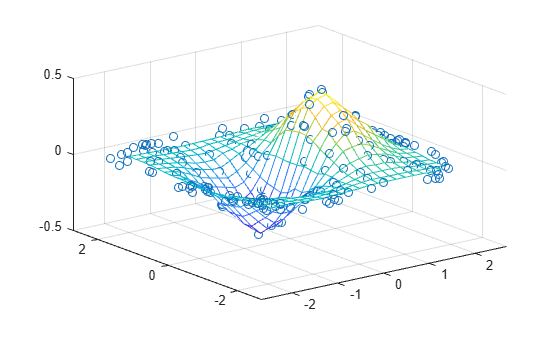Interpolate a 3-D slice of a 4-D function that is sampled at randomly scattered points.

Sample a 4-D function $\mathit{v}\left(\mathit{x},\mathit{y},\mathit{z}\right)$ at 2500 random points between `-1` and `1`. The vectors `x`, `y`, and `z` contain the nonuniform sample points.

```x = 2*rand(2500,1) - 1; y = 2*rand(2500,1) - 1; z = 2*rand(2500,1) - 1; v = x.^2 + y.^3 - z.^4;```

Create a grid with xy points in the range [-1, 1], and set $\mathit{z}=0$. Interpolating on this grid of 2-D query points `(xq,yq,0)` produces a 3-D interpolated slice `(xq,yq,0,vq)` of the 4-D data set `(x,y,z,v)`.

```d = -1:0.05:1; [xq,yq,zq] = meshgrid(d,d,0);```

Interpolate the scattered data on the grid. Plot the results.

```vq = griddata(x,y,z,v,xq,yq,zq); plot3(x,y,v,"ro") hold on surf(xq,yq,vq) hold off```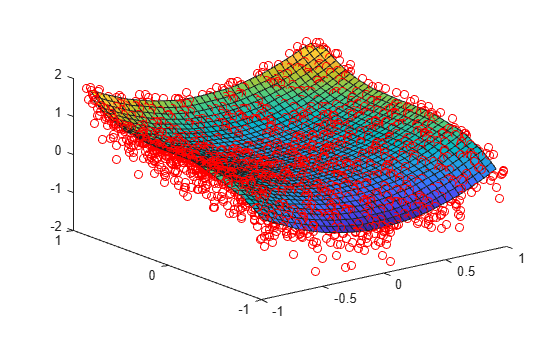Compare the results of several different interpolation algorithms offered by `griddata`.

Create a sample data set of 50 scattered points. The number of points is artificially small to highlight the differences between the interpolation methods.

```x = -3 + 6*rand(50,1); y = -3 + 6*rand(50,1); v = sin(x).^4 .* cos(y);```

Create a grid of query points.

`[xq,yq] = meshgrid(-3:0.1:3);`

Interpolate the sample data using the `"nearest"`, `"linear"`, `"natural"`, and `"cubic"` methods. Plot the results for comparison.

```z1 = griddata(x,y,v,xq,yq,"nearest"); plot3(x,y,v,"mo") hold on mesh(xq,yq,z1) title("Nearest Neighbor") legend("Sample Points","Interpolated Surface","Location","NorthWest")```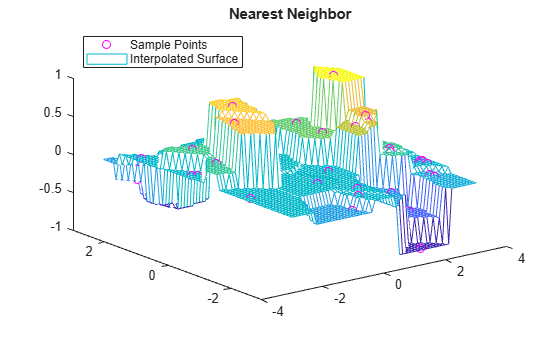```z2 = griddata(x,y,v,xq,yq,"linear"); figure plot3(x,y,v,"mo") hold on mesh(xq,yq,z2) title("Linear") legend("Sample Points","Interpolated Surface","Location","NorthWest")```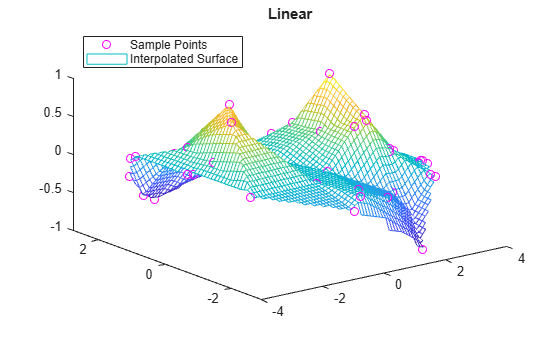```z3 = griddata(x,y,v,xq,yq,"natural"); figure plot3(x,y,v,"mo") hold on mesh(xq,yq,z3) title("Natural Neighbor") legend("Sample Points","Interpolated Surface","Location","NorthWest")```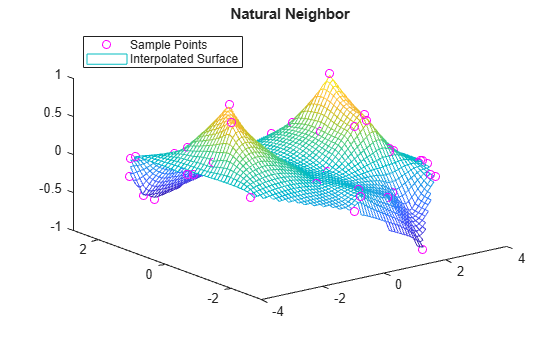```z4 = griddata(x,y,v,xq,yq,"cubic"); figure plot3(x,y,v,"mo") hold on mesh(xq,yq,z4) title("Cubic") legend("Sample Points","Interpolated Surface","Location","NorthWest")```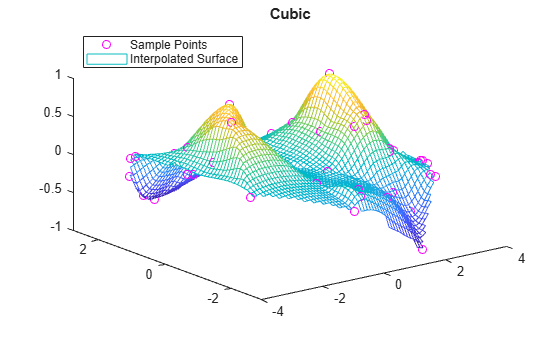Plot the exact solution.

```figure plot3(x,y,v,"mo") hold on mesh(xq,yq,sin(xq).^4 .* cos(yq)) title("Exact Solution") legend("Sample Points","Exact Surface","Location","NorthWest")```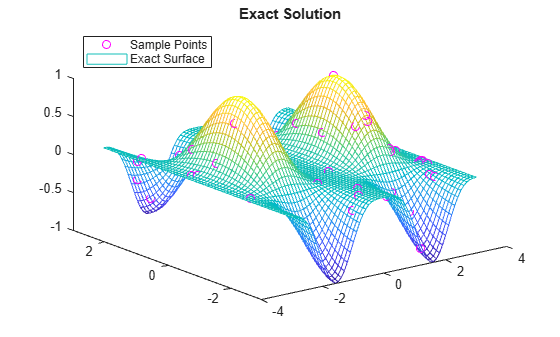## Input Arguments

collapse all

Sample point coordinates, specified as vectors. Corresponding elements in `x`, `y`, and `z` specify the xyz coordinates of points where the sample values `v` are known. The sample points must be unique.

Data Types: `double`

Sample values, specified as a vector. The sample values in `v` correspond to the sample points in `x`, `y`, and `z`.

If `v` contains complex numbers, then `griddata` interpolates the real and imaginary parts separately.

Data Types: `double`
Complex Number Support: Yes

Query points, specified as vectors or arrays. Corresponding elements in the vectors or arrays specify the xyz coordinates of the query points. The query points are the locations where `griddata` performs interpolation.

The specified query points must lie inside the convex hull of the sample data points. `griddata` returns `NaN` for query points outside of the convex hull.

Data Types: `double`

Interpolation method, specified as one of the methods in this table.

MethodDescriptionContinuity
`"linear"`Triangulation-based linear interpolation (default) supporting 2-D and 3-D interpolation.C0
`"nearest"`Triangulation-based nearest neighbor interpolation supporting 2-D and 3-D interpolation.Discontinuous
`"natural"`Triangulation-based natural neighbor interpolation supporting 2-D and 3-D interpolation. This method is an efficient tradeoff between linear and cubic.C1 except at sample points
`"cubic"`Triangulation-based cubic interpolation supporting 2-D interpolation only.C2
`"v4"`

Biharmonic spline interpolation (MATLAB® 4 `griddata` method) supporting 2-D interpolation only. Unlike the other methods, this interpolation is not based on a triangulation.

C2

Data Types: `char` | `string`

## Output Arguments

collapse all

Interpolated values, returned as a vector or array. The size of `vq` depends on the size of the query point inputs `xq`, `yq`, and `zq`:

• For 2-D interpolation, where `xq` and `yq` specify an `m`-by-`n` grid of query points, `vq` is an `m`-by-`n` array.

• For 3-D interpolation, where `xq`, `yq`, and `zq` specify an `m`-by-`n`-by-`p` grid of query points, `vq` is an `m`-by-`n`-by-`p` array.

• If `xq`, `yq`, (and `zq` for 3-D interpolation) are vectors that specify scattered points, then `vq` is a vector of the same length.

For all interpolation methods other than `"v4"`, the output `vq` contains `NaN` values for query points outside the convex hull of the sample data. The `"v4"` method performs the same calculation for all points regardless of location.

Grid coordinates for query points, returned as vectors or matrices. The shape of `Xq` and `Yq` depends on how you specify `xq` and `yq`:

• If you specify `xq` as a row vector and `yq` as a column vector, then `griddata` uses those grid vectors to form a full grid with ```[Xq,Yq] = meshgrid(xq,yq)```. In this case, the `Xq` and `Yq` outputs are returned as matrices that contain the full grid coordinates for the query points.

• If `xq` and `yq` are both row vectors or both column vectors, then ```Xq = xq``` and `Yq = yq`.

## Version History

Introduced before R2006a